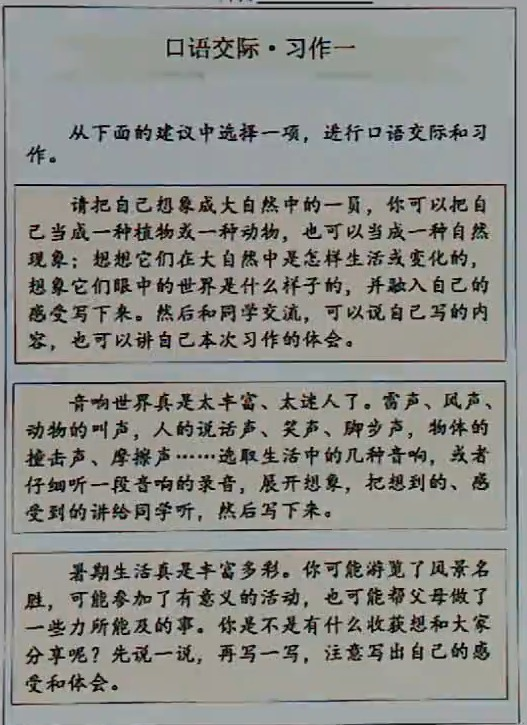10:12:59 五年级 语文 学生：137****8214

`                                             口语交际·习作一      从下面的建议中选择一项,进行口语交际和习作。      请把自己想象成大白然中的一员,你可以把自己当成一种植物或一种动物,也可以当成一种自然现象;想想它们在大自然中是怎样生活或变化的想象它们眼中的世界是什么样子的,并融入自己的感受写下来。然后和同学交流,可以说自己写的内容,也可以讲自己本次习作的体会。      音响世界真是太丰富、太迷人了。雷声、风声动物的叫声,人的说话声、笑声、脚步声,物体的撞击声、摩擦声……选取生活中的几种音响,或者仔细听一段音响的录音,展开想象,把想到的、感受到的讲给同学听,然后写下来。     暑期生活真是丰富多彩。你可能游览了风景名胜,可能参加了有意义的活动,也可能帮父母做了些力所能及的事。你是不是有什么收获想和大家分享呢?先说一说,再写一写,注意写出自己的感受和体会。`10:24:55 老师：183****6301

• 约等于
• 小于
• 大于
• ÷

除号
• 求积符号
• 并符号
• 交符号
• 属于
• 因为
• 所以
• 垂直于
• 平行线符号
• 弧线
• 波浪号
• 全等于
• 相似于
• 根号
• 小于等于
• 大于等于
• 不小于号
• 不大于号
• 证明号
• ±

正负号
• 求和符号
• ×

乘号
• 对数符号
• 公差符号
• 斜三角形
• 对数符
• 不等于号
• 小于等于
• 小于等于
• 平方米
• 摄氏度
• 公斤符号
• 千分号
• 百分号
• 恒等于
• 立方米
• 无限大号
• 圆周
• 三角形
• 单价
• 积分
• |x|

绝对值

• 约等于
• 小于
• 大于
• ÷

除号
• 求积符号
• 并符号
• 交符号
• 属于
• 因为
• 所以
• 垂直于
• 平行线符号
• 弧线
• 波浪号
• 全等于
• 相似于
• 根号
• 小于等于
• 大于等于
• 不小于号
• 不大于号
• 证明号
• ±

正负号
• 求和符号
• ×

乘号
• 对数符号
• 公差符号
• 斜三角形
• 对数符
• 不等于号
• 小于等于
• 小于等于
• 平方米
• 摄氏度
• 公斤符号
• 千分号
• 百分号
• 恒等于
• 立方米
• 无限大号
• 圆周
• 三角形
• 单价
• 积分
• |x|

绝对值

• 约等于
• 小于
• 大于
• ÷

除号
• 求积符号
• 并符号
• 交符号
• 属于
• 因为
• 所以
• 垂直于
• 平行线符号
• 弧线
• 波浪号
• 全等于
• 相似于
• 根号
• 小于等于
• 大于等于
• 不小于号
• 不大于号
• 证明号
• ±

正负号
• 求和符号
• ×

乘号
• 对数符号
• 公差符号
• 斜三角形
• 对数符
• 不等于号
• 小于等于
• 小于等于
• 平方米
• 摄氏度
• 公斤符号
• 千分号
• 百分号
• 恒等于
• 立方米
• 无限大号
• 圆周
• 三角形
• 单价
• 积分
• |x|

绝对值